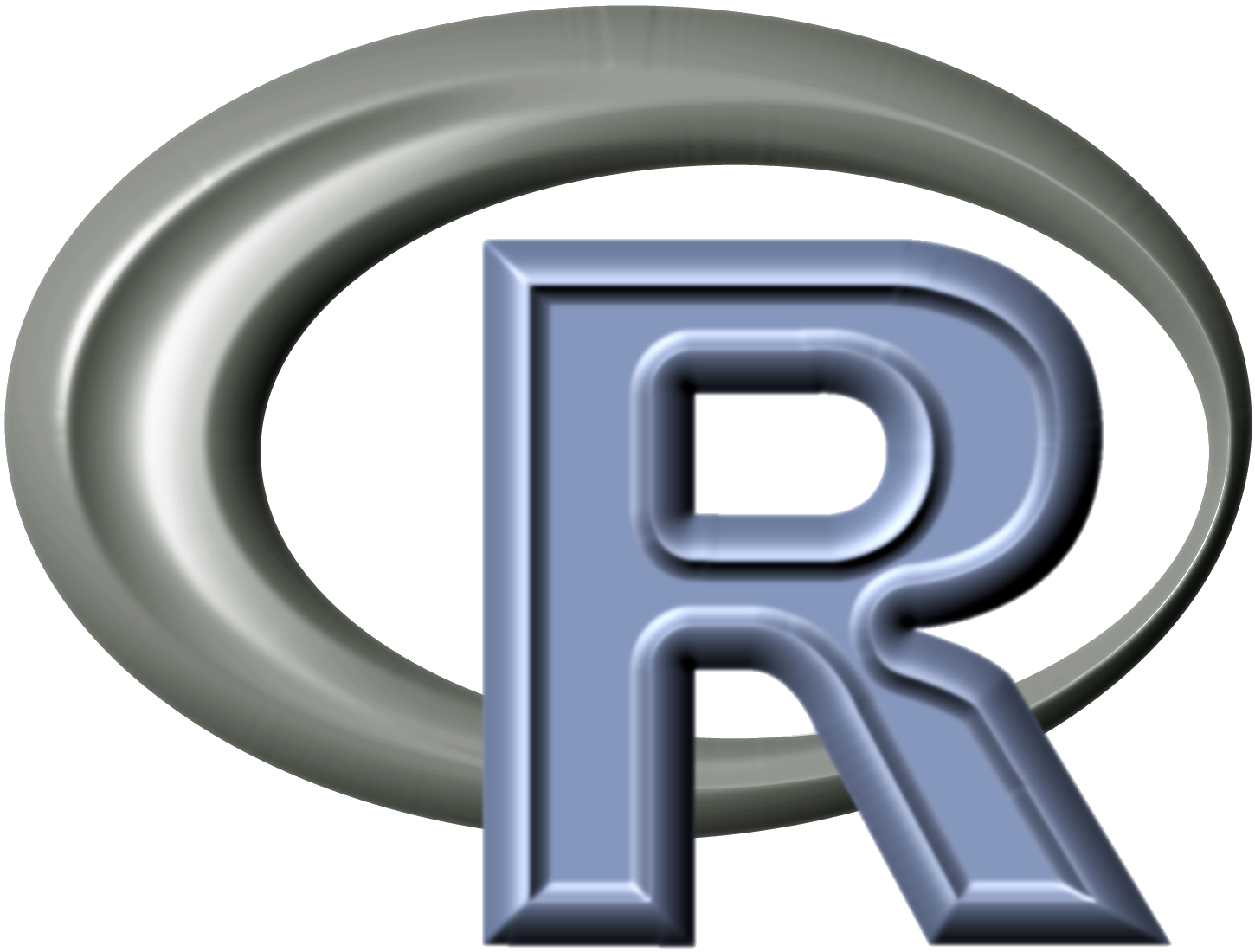# Good Ways to Do Scientific Computing

1. Break down the work into smaller modules and develop pipelines (consists of module) for the work. Be sure to save (important) intermediate results so that you can resume failed modules withouting reruning succeeded ones.

2. Manage your project in GitHub and use issues to manage tasks to do and their priorities …

# Estimation of False Discovery Rate using Sequential Permutation PvaluesI wrote a paper on sequential permutation test with Tim Bancroft and Dan Nettleton. The paper "T. Bancroft, C. Du and D. Nettleton (2012). Estimation of False Discovery Rate Using Sequential Permutation P­Values." has been accepted by Biometrics. To illustrate ideas in the paper and make sequential permutation test …

# Some Useful Properties of Modified Bessel Functions of the First Kind

I have to deal with Modified Bessel functions of the first kind frequently in my research. Here I list some useful properties of them for future reference.

$$I_0'(x) = I_1(x)$$
$$I_1'(x) = \frac{I_0(x)+I_2(x)}{2}$$
\frac{I_1(x)}{I_0(x)}\uparrow \Leftrightarrow I_0(x)(I_0(x …